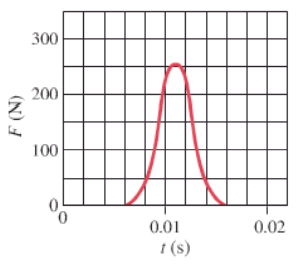×
Get Full Access to Physics: Principles With Applications - 6 Edition - Chapter 7 - Problem 20p
Get Full Access to Physics: Principles With Applications - 6 Edition - Chapter 7 - Problem 20p

×

# (III) Suppose the force acting on a tennis ball (massISBN: 9780130606204 3

## Solution for problem 20P Chapter 7

Physics: Principles with Applications | 6th Edition

• Textbook Solutions
• 2901 Step-by-step solutions solved by professors and subject experts
• Get 24/7 help from StudySoup virtual teaching assistantsPhysics: Principles with Applications | 6th Edition

4 5 1 405 Reviews
15
5
Problem 20P

(III) Suppose the force acting on a tennis ball (mass 0.060 kg) points in the +x direction and is given by the graph of Fig. 7-33 as a function of time, (a) Use graphical methods (count squares) to estimate the total impulse given the ball, (b) Estimate the velocity of the ball after being struck, assuming the ball is being served so it is nearly at rest initially.FIGURE 7-33

Problem 20.

Step-by-Step Solution:

Solution 20P:

We have to calculate the value of total impulse imparted to the ball using graphical method and the final velocity of the ball.

Step 1 of 4

Concept:

Impulseof a force is equal to the product of forceand the time intervalfor which the force acts on the body. It is also equal to the change in momentumproduced in the body of mass, having initial and final velocitiesrespectively. Mathematically,Impulse can be also obtained in graphical method. The area under force and time curve gives the value of impulse acting on the given body.

Step 2 of 4

Step 3 of 4

##### ISBN: 9780130606204

The full step-by-step solution to problem: 20P from chapter: 7 was answered by , our top Physics solution expert on 03/03/17, 03:53PM. This full solution covers the following key subjects: ball, estimate, given, Being, Impulse. This expansive textbook survival guide covers 35 chapters, and 3914 solutions. Physics: Principles with Applications was written by and is associated to the ISBN: 9780130606204. The answer to “?(III) Suppose the force acting on a tennis ball (mass 0.060 kg) points in the +x direction and is given by the graph of Fig. 7-33 as a function of time, (a) Use graphical methods (count squares) to estimate the total impulse given the ball, (b) Estimate the velocity of the ball after being struck, assuming the ball is being served so it is nearly at rest initially. FIGURE 7-33 20.” is broken down into a number of easy to follow steps, and 71 words. Since the solution to 20P from 7 chapter was answered, more than 1889 students have viewed the full step-by-step answer. This textbook survival guide was created for the textbook: Physics: Principles with Applications, edition: 6.

Unlock Textbook Solution